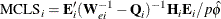### Case Deletion Diagnostic Statistics

For ordinary generalized linear models, regression diagnostic statistics developed by Williams (1987) can be requested in an output data set or in the OBSTATS table by specifying the DIAGNOSTICS | INFLUENCE option in the MODEL statement. These diagnostics measure the influence of an individual observation on model fit, and generalize the one-step diagnostics developed by Pregibon (1981) for the logistic regression model for binary data.

Preisser and Qaqish (1996) further generalized regression diagnostics to apply to models for correlated data fit by generalized estimating equations (GEEs), where the influence of entire clusters of correlated observations, or the influence of individual observations within a cluster, is measured. These diagnostic statistics can be requested in an output data set or in the OBSTATS table if a model for correlated data is specified with a REPEATED statement.

The next two sections use the following notation: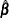is the maximum likelihood estimate of the regression parameters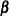, or, in the case of correlated data, the solution of the GEEs.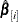is the corresponding estimate evaluated with the ith observation deleted, or, in the case of correlated data, with the ith cluster deleted.

p

is the dimension of the regression parameter vector.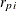is the standardized Pearson residual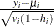, where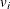is the variance of the ith response and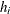is the leverage defined in the section H |LEVERAGE.is the variance of response i,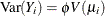, where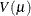is the variance function andis the dispersion parameter.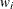is the prior weight of the ith observation specified with the WEIGHT statement. If there is no WEIGHT statement,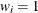for all i.

All unknown quantities are replaced by their estimated values in the following two sections.

#### Diagnostics for Ordinary Generalized Linear Models

The following statistics are available for generalized linear models.

##### DFBETA

The DFBETA statistic for measuring the influence of the ith observation is defined as the one-step approximation to the difference in the MLE of the regression parameter vector and the MLE of the regression parameter vector without the ith observation. This one-step approximation assumes a Fisher scoring step, and is given by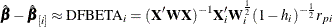whereis the leverage defined in the section H |LEVERAGE.

##### DFBETAS

The standardized DFBETA statistic for assessing the influence of the ith observation on the jth regression parameter is defined as the DFBETA statistic for the jth parameter divided by its estimated standard deviation, where the standard deviation is estimated from all the data.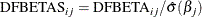##### DOBS |COOKD |COOKSD

In normal linear regression, the influence of observation i can be measured by Cook’s distance (Cook and Weisberg, 1982). A measure of influence of observation i for generalized linear models that is equivalent to Cook’s distance for normal linear regression is given by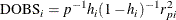whereis the leverage defined in the section H |LEVERAGE. This measure is the one-step approximation to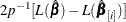, where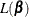is the log likelihood evaluated at.

##### H |LEVERAGE

The Fisher scores, or expected, weight for observation i is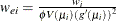. Let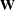be the diagonal matrix with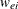as the ith diagonal. The leverageof the ith observation is defined as the ith diagonal element of the hat matrix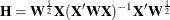#### Diagnostics for Models Fit by Generalized Estimating Equations (GEEs)

The diagnostic statistics in this section were developed by Preisser and Qaqish (1996). See the section Generalized Estimating Equations for further information and notation for generalized estimating equations (GEEs). The following additional notation is used in this section.

Partition the design matrix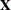and response vector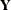by cluster; that is, let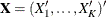, and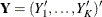corresponding to the K clusters.

Let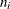be the number of responses for cluster i, and denote by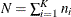the total number of observations. Denote by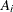the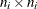diagonal matrix with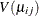as the jth diagonal element. If there is a WEIGHT statement, the diagonal element ofis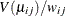, where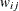is the specified weight of the jth observation in the ith cluster. Let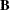the
diagonal matrix with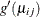as diagonal elements,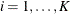,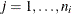. Let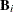thediagonal matrix corresponding to cluster i withas the jth diagonal element.

Letbe the
block diagonal weight matrix whose ith block, corresponding to the ith cluster, is thematrix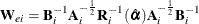where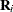is the working correlation matrix for cluster i.

Let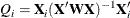where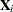is the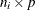design matrix corresponding to cluster i.

Define the adjusted residual vector as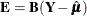and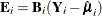, the estimated residual for the ith cluster.

Let the subscript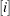denote estimates evaluated without the ith cluster,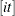estimates evaluated using all the data except the tth observation of the ith cluster, and let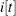denote matrices corresponding to the ith cluster without the tth observation.

The following statistics are available for generalized estimating equation models.

##### CH |CLUSTERH |CLEVERAGE

The leverage of cluster i is contained in the matrix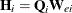, and is summarized by the trace of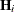,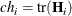The leverageof the tth observation in the ith cluster is the tth diagonal element of.

##### DFBETAC

The effect of deleting cluster i on the estimated parameter vector is given by the following one-step approximation for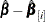: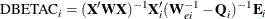##### DFBETACS

The cluster deletion statistic DFBETAC can be standardized using the variances ofbased on the complete data. The standardized one-step approximation for the change in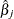due to deletion of cluster i is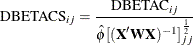##### DFBETAO

Partition the matrices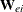and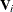as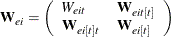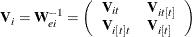and let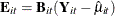and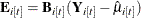.

The effect of deleting the tth observation from the ith cluster is given by the following one-step approximation to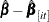: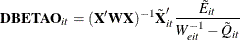where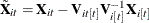,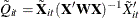, and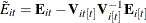. Note that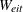,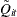, and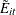are scalars.

##### DFBETAOS

The observation deletion statistic DFBETAO can be standardized using the variances ofbased on the complete data. The standardized one-step approximation for the change indue to deletion of observation t in cluster i is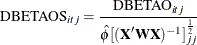##### DCLS |CLUSTERCOOKD |CLUSTERCOOKSD

A measure of the standardized influence of the subset m of observations on the overall fit is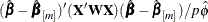. For deletion of cluster i, this is approximated by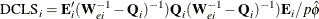##### DOBS |COOKD |COOKSD

The measure of overall fit in the section DCLS |CLUSTERCOOKD |CLUSTERCOOKSD for the deletion of the tth observation in the ith cluster is approximated by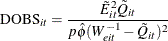where,, andare defined in the section DFBETAO. In the case of the independence working correlation, this is equal to the measure for ordinary generalized linear models defined in the section DOBS |COOKD |COOKSD.

##### MCLS |CLUSTERDFIT

A studentized distance measure of the type defined in the section DCLS |CLUSTERCOOKD |CLUSTERCOOKSD of the influence of the ith cluster is given by